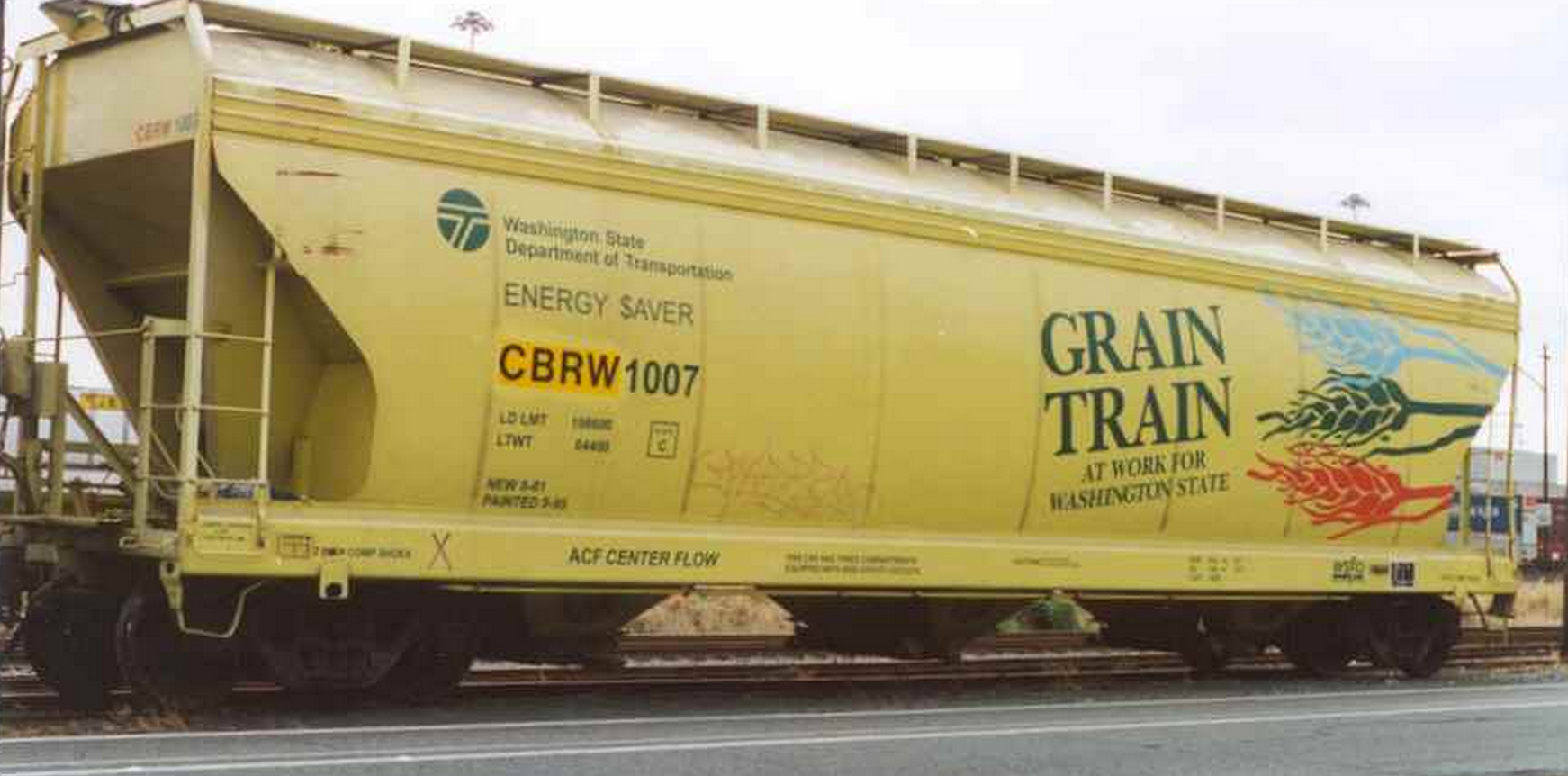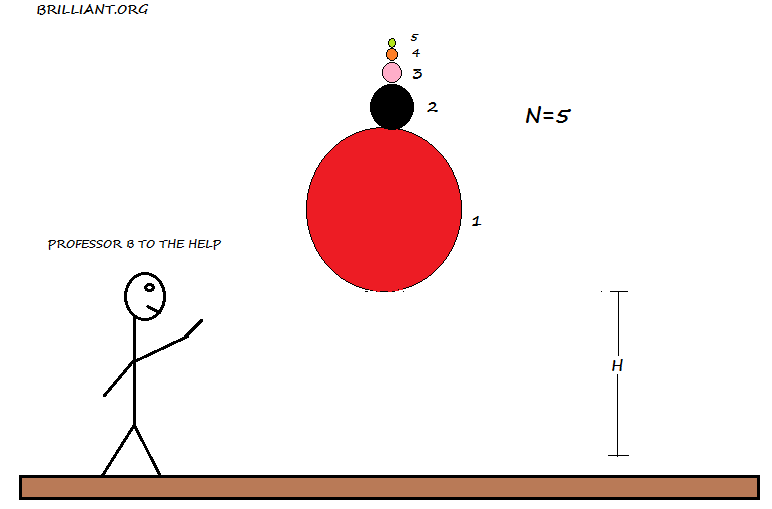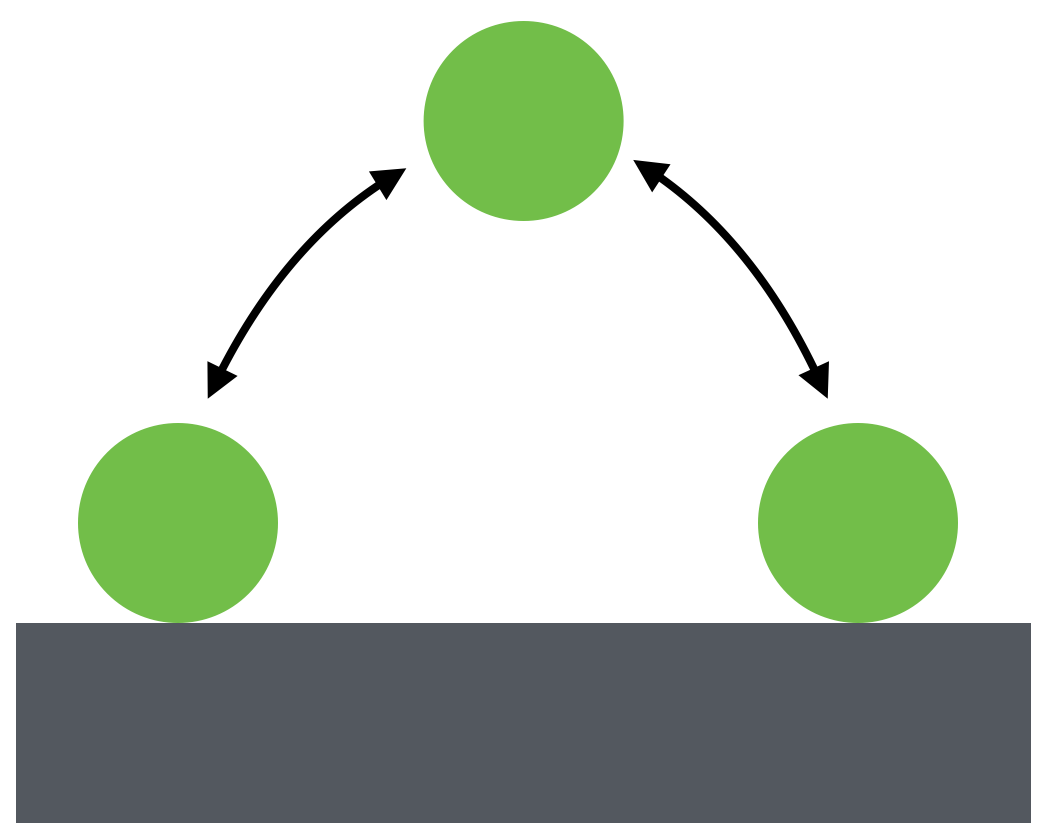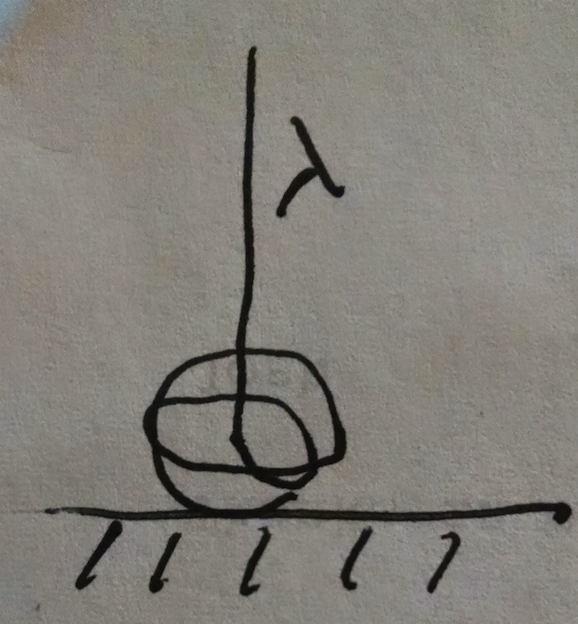Classical Mechanics

# Momentum: Level 3-4 Challenges

You want to pour exactly $300 \text{ g}$ of sugar into a bowl. You place the bowl on a scale and zero it. Then you pour sugar, from a constant height at a constant rate. When should you stop pouring to ensure that you have exactly $300\text{ g}$ of sugar?

Details and Assumptions:

• Ignore air resistance during the motion.

• Pouring means that the sugar initially has zero velocity.

• Once the sugar reaches the scale, it is brought to a full stop.

• The time needed to pour the 300 g of sugar is longer than the falling time of the sugar.

Bonus: Will the answer change if the sugar bounces up and down a bit before finally settling?Grain is loaded onto a train initially coasting at the speed $v_0 \text{ m/s}$ by dropping it vertically from a stationary silo at the rate $\alpha_m \text{ kg/s}$. How fast is the train moving when it exits from under the silo (in m/s)?

Details

• The train is $l = 200$ m long, and has an empty weight of $m_0 = 500$ kg.
• $v_0=45$ m/s
• $\alpha_m = 20$ kg/sGrain is loaded onto a train initially coasting at the speed $v_0 \text{ m/s}$ by dropping it vertically from a stationary silo at the rate $\alpha_m \text{ kg/s}$. How long does it take for the train to pass the silo (in seconds)?

Details

• The train is $l = 200$ m long, and has an empty weight of $m_0 = 500$ kg.
• $v_0=45$ m/s
• $\alpha_m = 20$ kg/sBouncy balls are stacked on top of each other with decreasing mass as shown In the figure above. They are configured such that the one on the bottom is at a height of $H$ above the ground. The figure above shown for $n=5$, but we can increase the number of ball to our liking.

After the balls are released, and the first ball touches the ground, each ball bounces off its neighboring ball. If $v_{n}$ is the speed of the $nth$ ball right after it bounces of its adjacent ball. What is the ratio of the speed of the $20th$ ball right after it bounces to the $5th$ ball right after it bounces.

Simply put what is $\large{\frac { { v }_{ 20 } }{ { v }_{ 5 } }}$

Details and assumptions

All the collisions that take place are elastic collisions.

Assume ${ M }_{ 1 }\gg { M }_{ 2 }\gg { M }_{ 3 }\gg ........{ M }_{ n }$.Suppose you want to throw a super ball so that it bounces back and forth, retracing the same trajectory, as shown above. If you throw the ball horizontally with velocity $\SI[per-mode=symbol]{1}{\meter\per\second},$ what spin $\omega$ (in $\si[per-mode=symbol]{\radian\per\second}$) must you give it so that it does what you want?

Assumptions and Details

• The collision with the ground is perfectly elastic.
• The ball does not slip while it contacts the ground.
• The radius of the ball is $\SI{3}{\centi\meter}.$A 100 m rope of line density $\lambda=0.1$ kg/m is suspended vertically so that the bottom of the rope is just touching a scale. It is then released and falls onto the scale, which perceives a time dependent weight.

What is the reading on the scale in Newtons at the moment half the rope lies on the scale?

Assumptions and Details

• $g=9.8$ m/s$^{2}$.
×